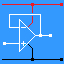RANDOM PAGE

SITE SEARCH

LOG
IN

HELP

# Decibels

This is the AQA version closing after June 2019. Visit the the version for Eduqas instead.

## Decibels - The Basics

• Decibels are used to compare one signal with another.
• 0 dB is the quietest sound a typical or average human can hear.
• You can say that one signal is 20dB stronger that the other. The signal voltage would be 10 times greater.
• You can say that one signal is 40dB stronger that the other. The signal voltage would be 100 times greater.
• You can say that one signal is 60dB stronger that the other. The signal voltage would be 1000 times greater.
• Note that adding 20dB multiplies the voltage by 10.
• Decibels are often used to compare the wanted signal with the unwanted background noise.
• Sound levels are quoted in dB. This is how loud the sound is compared with a reference sound level.
• Useful dB Examples on Wikipedia

## A2 - Decibels and Signal To Noise Ratio (S/N or SNR)

• For voltage measurements:        SNR = 20 log10(VS/VN)
• For power measurements:        SNR = 10 log10(PS/PN)
• SNR is the signal to noise ratio
• VS is the signal voltage
• VN is the noise voltage
• PS is the signal power
• PN is the noise power
• SNR is measured in dB (Decibels)
• For noise free TV reception (no snow on the picture) the SNR needs to be 50dB.
• Speech becomes unintelligible if the SNR is less than about 10 dB.

## Logarithmic Scales

Signal to noise ratio is measured in decibels. This is because received signals can be 1,000,000,000 times weaker than transmitted signals. The decibel scale is ideal for representing such a huge range of values. The decibel scale is logarithmic. A huge range of numbers is reduced to small manageable range.

Base 10 logarithms work like this ...

 Number Log 10 0.001 -3 0.01 -2 0.1 -1 1 0 10 1 100 2 1000 3 10000 4 100000 5 1000000 6

## Examples

### dB Example 1

• Signal level is 50µV
• Noise level is 1µV
• SNR = 20 log10(50/1)
• SNR = 20 x 1.7
• SNR = +34dB
• This is a nice strong signal with a little noise in the background.

### dB Example 2

• Signal level is 1µV
• Noise level is 1µV
• SNR = 20 log10(1/1)
• SNR = 20 x 0
• SNR = 0dB
• This is a very weak signal with the signal almost disappearing into the noise.

### dB Example 3

• Signal level is 0.1µV
• Noise level is 1µV
• SNR = 20 log10(0.1/1)
• SNR = 20 x -1
• SNR = -20dB
• The negative SNR indicates that the signal is completely swamped by the noise.

reviseOmatic V3     Contacts, ©, Cookies, Data Protection and Disclaimers Hosted at linode.com, London